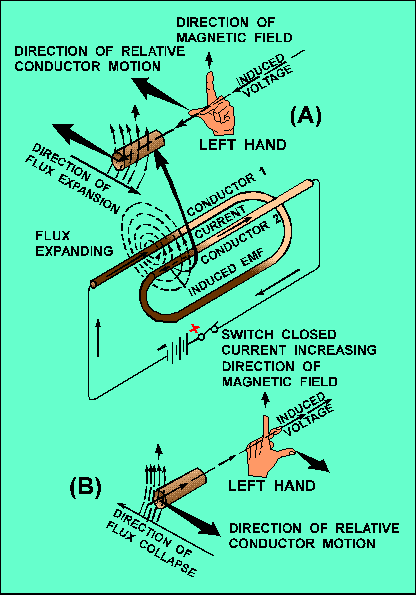Self-Inductance inductance. As you know, current in a conductor produces a magnetic field surrounding the conductor. When the current changes, the magnetic field changes. This causes relative motion between the magnetic field and the conductor, and an electromotive force (emf) is induced in the conductor. This emf is called a SELF-INDUCED EMF because it is induced in the conductor carrying the current.">Custom SearchSELF-INDUCTANCE Even a perfectly straight length of conductor has some inductance. As you know, current in a conductor produces a magnetic field surrounding the conductor. When the current changes, the magnetic field changes. This causes relative motion between the magnetic field and the conductor, and an electromotive force (emf) is induced in the conductor. This emf is called a SELF-INDUCED EMF because it is induced in the conductor carrying the current. The emf produced by this moving magnetic field is also referred to as COUNTER ELECTROMOTIVE FORCE (cemf). The polarity of the counter electromotive force is in the opposite direction to the applied voltage of the conductor. The overall effect will be to oppose a change in current magnitude. This effect is summarized by Lenz's law which states that: THE INDUCED EMF IN ANY CIRCUIT IS ALWAYS IN A DIRECTION TO OPPOSE THE EFFECT THAT PRODUCED IT. If the shape of the conductor is changed to form a loop, then the electromagnetic field around each portion of the conductor cuts across some other portion of the same conductor. This is shown in its simplest form in figure 2-2. A length of conductor is looped so that two portions of the conductor lie next to each other. These portions are labeled conductor 1 and conductor 2. When the switch is closed, current (electron flow) in the conductor produces a magnetic field around ALL portions of the conductor. For simplicity, the magnetic field (expanding lines of flux) is shown in a single plane that is perpendicular to both conductors. Although the expanding field of flux originates at the same time in both conductors, it is considered as originating in conductor 1 and its effect on conductor 2 will be explained. With increasing current, the flux field expands outward from conductor 1, cutting across a portion of conductor 2. This results in an induced emf in conductor 2 as shown by the dashed arrow. Note that the induced emf is in the opposite direction to (in OPPOSITION to) the battery current and voltage, as stated in Lenz's law. Figure 2-2. - Self-inductance.The direction of this induced voltage may be determined by applying the LEFT-HAND RULE FOR GENERATORS. This rule is applied to a portion of conductor 2 that is "lifted" and enlarged for this purpose in figure 2-2(A). This rule states that if you point the thumb of your left hand in the direction of relative motion of the conductor and your index finger in the direction of the magnetic field, your middle finger, extended as shown, will now indicate the direction of the induced current which will generate the induced voltage (cemf) as shown. In figure 2-2(B), the same section of conductor 2 is shown after the switch has been opened. The flux field is collapsing. Applying the left-hand rule in this case shows that the reversal of flux MOVEMENT has caused a reversal in the direction of the induced voltage. The induced voltage is now in the same direction as the battery voltage. The most important thing for you to note is that the self-induced voltage opposes BOTH changes in current. That is, when the switch is closed, this voltage delays the initial buildup of current by opposing the battery voltage. When the switch is opened, it keeps the current flowing in the same direction by aiding the battery voltage. Thus, from the above explanation, you can see that when a current is building up it produces an expanding magnetic field. This field induces an emf in the direction opposite to the actual flow of current. This induced emf opposes the growth of the current and the growth of the magnetic field. If the increasing current had not set up a magnetic field, there would have been no opposition to its growth. The whole reaction, or opposition, is caused by the creation or collapse of the magnetic field, the lines of which as they expand or contract cut across the conductor and develop the counter emf. Since all circuits have conductors in them, you can assume that all circuits have inductance. However, inductance has its greatest effect only when there is a change in current. Inductance does NOT oppose current, only a CHANGE in current. Where current is constantly changing as in an ac circuit, inductance has more effect. Q.3 Define inductance. Q.4 What is meant by induced emf? By counter emf? Q.5 State Lenz's law. Q.6 What effect does inductance have (a) on steady direct current and (b) on direct current while it is changing in amplitude?

Privacy Statement - Copyright Information. - Contact UsIntegrated Publishing, Inc.